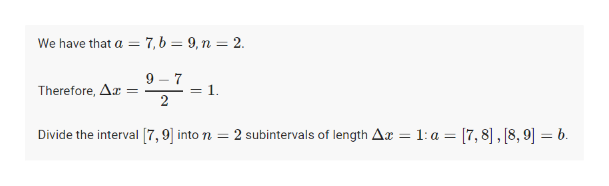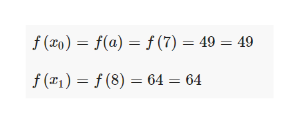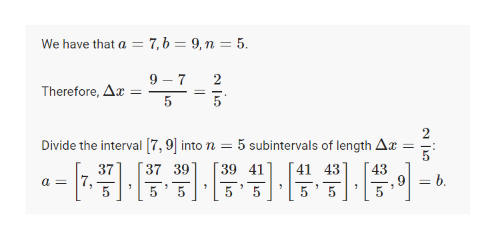# Let f(x) = x2 and compute the Riemann sum of f over the interval [7,9], using the following number of subintervals (n). In each case, choose the representative points to be the right endpoints of the subintervals. (Round your answers to two decimal places.)(a) Use two subintervals of equal length (n = 2). (b) Use five subintervals of equal length (n = 5). (c) Use ten subintervals of equal length (n = 10).

Question
55 views

Let f(x) = x2 and compute the Riemann sum of f over the interval [7,9], using the following number of subintervals (n). In each case, choose the representative points to be the right endpoints of the subintervals. (Round your answers to two decimal places.)

(a) Use two subintervals of equal length (n = 2).

(b) Use five subintervals of equal length (n = 5).

(c) Use ten subintervals of equal length (n = 10).

check_circle

Step 1

Find the intervals first.help_outlineImage Transcriptionclose7,b = 9,n = 2. We have that a 9 7 Therefore, A = 1. 2 Divide the interval 7,9] into n 2 subintervals of length Ax = 1: a = [7,8],[8,9] = b fullscreen
Step 2

Now, we evaluate the function at the left endpoints.

Finally,  sum up the above values and multiply by delta x=1

1(49+64)=113help_outlineImage Transcriptionclosef (x0) = f(a) f (7) = 49 = 49 f (r1)=f(8) 64 = 64 fullscreen
Step 3

b) Find the interv...help_outlineImage Transcriptionclose7,b = 9,n = 5 We have that a 9 7 51 2 Therefore, Ar Divide the interval [7,9] into n 5 subintervals of length Ax 39 41 41 43 37 39 37 a 7, 43 - 5 5 5 5 fullscreen

### Want to see the full answer?

See Solution

#### Want to see this answer and more?

Solutions are written by subject experts who are available 24/7. Questions are typically answered within 1 hour.*

See Solution
*Response times may vary by subject and question.
Tagged in

### Other